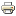Sales and technical support 07572 532 884# What are the different voltages in AC and what does RMS mean?Printer-friendly version

With AC the voltage is constantly changing and so as to get a single number to use for discussion and calculation purposes, engineers use the root of the mean of the square (RMS) voltage, or occasionally either the peak, or the peak-to-peak voltage.

With a pure sinusoidal waveform the voltage that is generally discussed is the RMS voltage because this is equivalent to the DC voltage that produces the same heating effect for a given current. So 240V RMS is equivalent to 339 V peak, or 679 V peak to peak and can be written as 240 Vrms. (the formula is Vrms = Vmax / √2).

The waveform is a sinusoid varying about a neutral, which can also be drawn as a vector with a single arrow pointing away from neutral. When a load is connected in an AC network the electrons oscillate backwards and forwards rather than flowing through it as they do in a DC network.

Broadly speaking current is related to voltage and resistance by the formula V=IR and so in a given system as voltage rises and falls so will current although there can be a time lag between the two as a result of capacitance and inductance in the circuit.